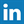# FreBlogg

## What is wattless current? | Target GATE 2015 | ECE | EEE | EIE

22:21 Posted by DurgaSwaroop
Watt-less Current?
Watt is the unit of power. So, whenever power is absorbed by an element it is measured in Watts.
So, for example, when a current flows through a Resistor, the Power is dissipated as heat. So, when current flows through a resistive element some power is dissipated, measured in Watts.

So, Now, as you might expect, Watt-less current is the current which doesn't dissipate any power. How, is that possible you ask, lets see how.
The Power in an AC Circuit is given by the following relation. P = V.I.Cos(Ø)
Ø, being the phase angle between Applied voltage and the Alternating Current.
The Quantity Cos(Ø) is also called as Power Factor.
The Phase angle, Ø, is zero for Resistors.
So, For a Resistor, P = V.I. Cos(0) = V.I (both are RMS Quantities)
But, when it comes to Capacitors and Inductors, the Voltage and the current are at angle of 90.

We know, Cos(90) = 0
So, For a pure Inductor or pure Capacitor , Power = 0.
So, even when the current flows in a Capacitor and Inductor, they don't dissipate any power. Such a Current is called "Watt-less Current",  because it won't consume any watts.
Capacitors and Inductors store the energy during the positive half-cycle and give it back during the negative half-cycle. So, they don't consume any power in the whole cycle.
Not so surprisingly, Capacitor and Inductor are called Watt-less Components.

For more articles like these, stay tuned to our blog by subscribing for our email notifications.
As always have a Happy reading and Good luck with your preparation!
-----------------------------------------------------------------------------------------------------------------------------
Author : Willtron
Used under Creative commons Licensing
------------------------------------------------------------------------------------------------------
Subscribe to our blog by entering your mail in the Get free updates! to your right, so that you won't miss any important post and also to be the first to get informed.
Folow us on our Facebook page @ Fre Blogg
Head over to my You Tube channel for some interesting tutorials @ You Tube